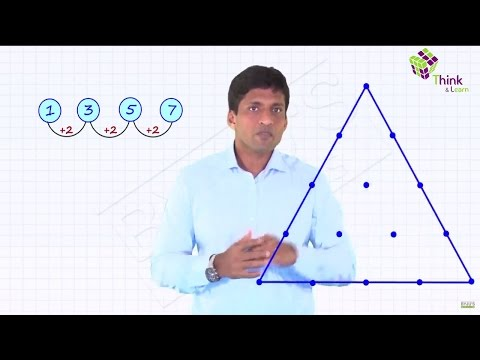# Arithmetic ProgressionsThe Arithmetic Progression is one of the important topics for CAT quantitative aptitude section. When a sequence falls in a pattern such that the difference between the consecutive numbers is constant, the numbers fall in an Arithmetic Progression or an AP.

For example 3, 6, 9, 12 form an arithmetic progression (AP) as the difference between consecutive numbers is 3. This AP has the first term as 3 and common difference as 3.

Similarly, 12, 8, 4, 0, -4 form another arithmetic progression (AP) with a common difference of –4. Here, the first term of AP is 12 and the common difference is -4.

Check this video to learn arithmetic progressions effectively and get complete notes on arithmetic progressions below.

## General form of an AP

Take an AP with the first term as ‘a’ and the common difference as ‘d’.

First term = a

Second term = a + d

Third term = a + 2d

Fourth term = a + 3d

So nth term = a + (n – 1) d

So, sum of arithmetic progression to first ‘n’ terms = a + a + d + a + 2d + a + 3d + a + 4d + … + a + (n – 1) d

= na + d + 2d + 3d + 4d + … + (n – 1) d

= na + d( 1 + 2 + 3 + … + (n – 1))

= na + d n(n-1)/2

(Taking n/2 common)= (n/2) ( 2a + (n-1)d)

=(n/2) ( a + a + (n – 1)d)

= (n/2) (first term + last term).

For an AP with first term ‘a’ and common difference ‘d’

• nth term : Tn = a + (n – 1)d
• Sum of arithmetic progression for first ‘n’ terms will be : Sn= n/2 (2a+(n-1)d) = (n/2) (first term + last term)

Average Of “n” Terms in an AP

If you observe the expression for sum of first ‘n’ terms= (n/2)(first term + last term)

You can write it as Sum= n/2(first term + last term)

Now, it is known that sum of ‘n’ terms = average × number of terms Here, number of terms = ‘n’

• Average of ‘n’ terms of an AP will be = (first term + last term)/2
• For an AP with first term ‘a’ and common difference ‘d’
• The middle point or (first term + last term)/2 is the average of all the terms.
• If we need to consider 3 consecutive terms in an AP, we take it as a-d, a and a + d
• Four consecutive terms in an AP; we take it as (a – 3d), (a – d), (a + d), (a + 3d)
• Five consecutive terms in an AP we will take it as (a – 2d),(a – d), a, (a + d), (a + 2d).

## Arithmetic Progression Examples:

1. How many terms are there in the AP? 20, 25, 30 … 130?

Solution:

First term ‘a’ = 20

Last term ‘T’ = 130

Common difference ‘d’ = 5

It is known that Tn = a + (n – 1)d

=>130 = 20 + 5( n – 1)

=>n – 1 = 110/5 = 22

=>n = 23

So there are 23 terms.

2.Find the value of expression 2 – 3 + 3 – 4 + 4 – 5 + … to 100 terms.

Solution:

If you look closely you realize that this expression is a combination of two progressions.

2 + 3 + 4 + … to 50 terms and 3 + 4 + 5 + … to 50 terms.

Now take 2 + 3 + … to 50 terms. It forms an AP with common difference 1.

Sum of 50 terms = (50/2) (2 + 51) = 1325

Take 3+4 + 5 + 6 + … to 50 terms.

It forms an AP with common difference 1. Sum of 50 terms = (50/2) (3 + 52) = 1375

So the answer is (2 + 3 + 4 + …) – (3+ 4 + 5 …) = 1325 – 1375 = – 50

You can also group two digits together to form another pattern

(2 – 3)+(3 – 4)…. = -1 – 1….50 times . i.e. for the addition of every two terms, the result will be (–1).

Since there are 50 such pairs, the total is -50 Sum= -50.

3. The sum of three numbers in an AP is 9 while the sum of there squares = 35. Find the numbers.

Solution:

Let the numbers be ‘a – d’, ‘a’ and ‘a + d’

Sum of these three numbers 3a = 9 ? a = 9/3 = 3

Sum of their squares = (a – d)2 + a2 + (a – d)2 = 35? 2d2 + 27 = 35 ? d = ± 2

When d = 2, the numbers are 1, 3, 5. When d = -2, the numbers are 5, 3, 1.

4. The sum of 3rd and 15th elements of an AP is equal to the sum of 6th, 11th and 13th elements of the same AP.Then which element of the series should necessarily be equal to zero?

a) 1st

b) 9th

c) 12th

d) none of these

Solution:

Tn = a + (n – 1)d.

T3 + T15 = T6 + T11 + T13 a + 2d + a + 14d = a + 5d + a + 10d + a + 12d

a – 16d + 27d = 0

a + 11d = 0

Hence, the 12th term will be 0.